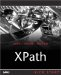Creating Arithmetic Expressions

 Besides primary expressions, XPath 2.0 also supports arithmetic operators for addition, subtraction, multiplication, division, and modulus . These operators are represented this way (note that instead of using the / symbol for division, which can be interpreted as markup, XPath 2.0 uses the div operator): + Addition - Subtraction and Negation * Multiplication div Division idiv Integer division mod Modulus USING THE SUBTRACTION OPERATOR In XPath 2.0, you must precede a subtraction operator with a space, because "-" is a legal name character. In other words, x-y can be interpreted as a name, whereas x - y is an expression involving a subtraction operation. The idiv operator requires its operands to be of type xs:integer and returns a result of type xs:integer , rounded toward zero. And the mod operator returns the remainder after a division (for example, 16 mod 3 is 1). Here are some examples of arithmetic expressions: 4 * 2 returns 8 33 + 11 returns 44 -5 div 2 returns 2.5 -5 idiv 2 returns 2 \$temp + 12 returns 72 if \$temp holds 60 -(20 + 6) returns 26 SUBTRACTING DATE VALUES In XPath 2.0, if you subtract two date values, the result is of the xdt:dayTimeDuration type.XPath Kick Start: Navigating XML with XPath 1.0 and 2.0
ISBN: 0672324113
EAN: 2147483647
Year: 2002
Pages: 131

Similar book on Amazon